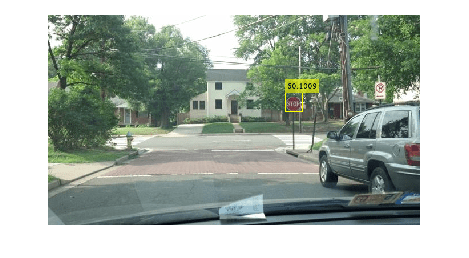# Generate Code for Detecting Objects in Images by Using ACF Object Detector

This example shows how to generate code from a MATLAB function that detects objects in images by using an `acfObjectDetector` object. When you intend to generate code from your MATLAB function that uses an `acfObjectDetector` object, you must create the object outside of the MATLAB function. The example explains how to modify the MATLAB code in Train Stop Sign Detector Using ACF Object Detector to support code generation.

### Design the MATLAB Code File for Code Generation

To generate C Code, MATLAB Coder requires MATLAB code to be in the form of a function. The arguments of the function cannot be MATLAB objects. This requirement presents a problem for generating code from the MATLAB function that uses `acfObjectDetector` objects created outside of the MATLAB function. To solve this problem, use the `toStruct` function to convert the `acfObjectDetector` object into a structure and pass the structure to the MATLAB function.

To support code generation, this example restructures the code of an existing example ( See Train Stop Sign Detector Using ACF Object Detector) in a function called `detectObjectsUsingACF`, which is present in the current working folder as a supporting file. The `detectObjectsUsingACF` function takes an image as an input and loads the pretrained ACF stop sign detector.

`type("detectObjectsUsingACF.m")`
```function [bboxes,scores] = detectObjectsUsingACF(InputImage) % Load a trained detector from a MAT file S = coder.load('detectorStruct.mat'); % Define a persistent variable persistent detector if isempty(detector) % Re-create the ACF Object Detector detector = acfObjectDetector(S.detectorStruct.Classifier,S.detectorStruct.TrainingOptions); end % Use the detect function to detect objects in the input image [bboxes,scores] = detect(detector,InputImage); end ```

### Create ACF Stop Sign Detector Outside of the MATLAB Function

`load("stopSignsAndCars.mat")`

Select the ground truth for stop signs. The ground truth data is the set of known locations of stop signs in the images.

`stopSigns = stopSignsAndCars(:,1:2);`

Add the full path to the image files.

```stopSigns.imageFilename = fullfile(toolboxdir("vision"),... "visiondata",stopSigns.imageFilename);```

Use the `trainACFObjectDetector` function to train the ACF detector. Turn off the training progress output by setting `Verbose=false`.

`detector = trainACFObjectDetector(stopSigns,NegativeSamplesFactor=2,Verbose=false); `

### Generate C-MEX Function

Because you intend to generate code for the MATLAB function `detectObjectsUsingACF`, convert the created `detector` into a structure.

`detectorStruct = toStruct(detector);`

Save the trained object structure as a MAT file.

`save("detectorStruct.mat","detectorStruct"); `

Generate C-MEX code that you can run in the MATLAB environment. Use the `codegen` (MATLAB Coder) command.

`codegen detectObjectsUsingACF -report -args { coder.typeof(uint8(0), [inf inf 3])}`
```Code generation successful: To view the report, open('codegen/mex/detectObjectsUsingACF/html/report.mldatx') ```

### Detect Objects Using Generated C-MEX Function

To detect objects in an image, load a test image.

`img = imread("stopSignTest.jpg");`

Call the generated C-MEX function by passing the loaded image `img` as an input.

`[bboxes,scores] = detectObjectsUsingACF_mex(img);`

Display the detection results and insert the bounding boxes for objects into the image.

```img = insertObjectAnnotation(img,"rectangle",bboxes,scores); figure imshow(img)```### Clean Up

Release the system memory used to store the generated C-MEX file.

`clear ObjectDetectionFromImages_mex;`